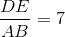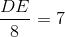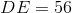# PSAT Math : How to find if right triangles are similar

## Example Questions

### Example Question #2 : Right Triangles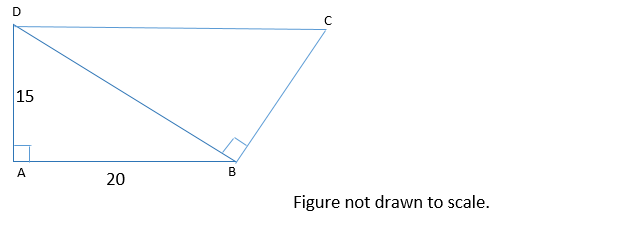In the figure above, line segments DC and AB are parallel. What is the perimeter of quadrilateral ABCD?

90

80

75

85

95

85

Explanation:

Because DC and AB are parallel, this means that angles CDB and ABD are equal. When two parallel lines are cut by a transversal line, alternate interior angles (such as CDB and ABD) are congruent.

Now, we can show that triangles ABD and BDC are similar. Both ABD and BDC are right triangles. This means that they have one angle that is the same—their right angle. Also, we just established that angles CDB and ABD are congruent. By the angle-angle similarity theorem, if two triangles have two angles that are congruent, they are similar. Thus triangles ABD and BDC are similar triangles.

We can use the similarity between triangles ABD and BDC to find the lengths of BC and CD. The length of BC is proportional to the length of AD, and the length of CD is proportional to the length of DB, because these sides correspond.

We don’t know the length of DB, but we can find it using the Pythagorean Theorem. Let a, b, and c represent the lengths of AD, AB, and BD respectively. According to the Pythagorean Theorem:

a2 + b2 = c2

152 + 202 = c2

625 = c2

c = 25

The length of BD is 25.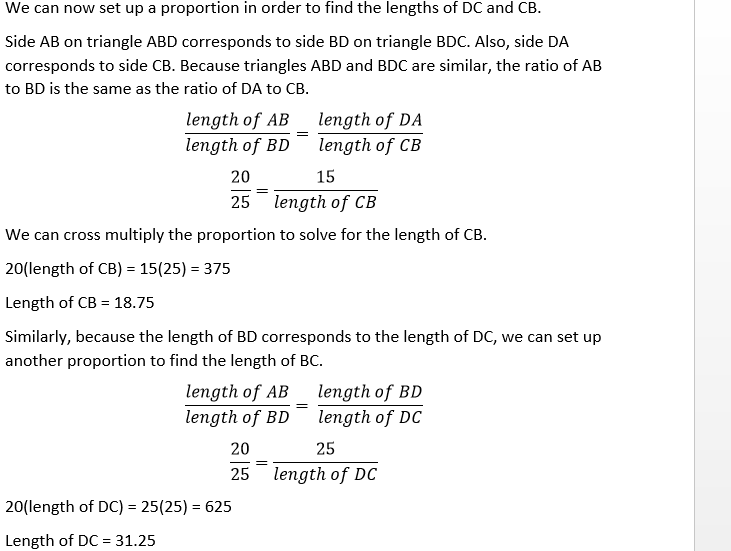We now have what we need to find the perimeter of the quadrilateral.

Perimeter = sum of the lengths of AB, BC, CD, and DA.

Perimeter = 20 + 18.75 + 31.25 + 15 = 85

### Example Question #1 : Right Triangles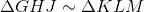and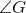is a right angle.

Which angle or angles must be complementary to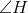?

I)II)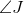III)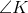IV)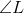V)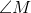II and V only

II only

IV only

I and III only

I only

II and V only

Explanation:is a right angle, and, since corresponding angles of similar triangles are congruent, so is. A right angle cannot be part of a complementary pair so both can be eliminated.can be eliminated, since it is congruent to; congruent angles are not necessarily complementary.

Sinceis right angle,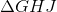is a right triangle, andandare its acute angles. That makescomplementary to. Sinceis congruent to, it is also complementary to.

The correct response is II and V only.

### Example Question #2 : Right Triangles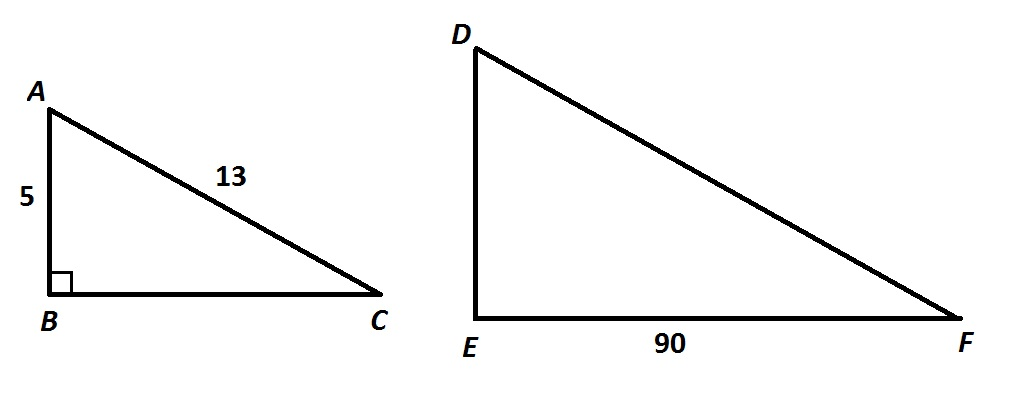Refer to the above figure. Given that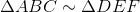, give the perimeter of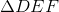.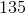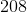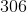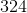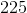Explanation:

By the Pythagorean Theorem,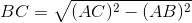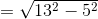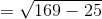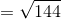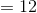The similarity ratio ofto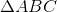is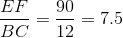which is subsequently the ratio of the perimeter ofto that of.

The perimeter ofis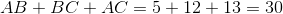,

so the perimeter ofcan be found using this ratio: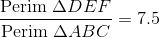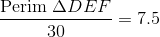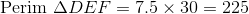### Example Question #3 : Right TrianglesNote: Figure NOT drawn to scale.

Refer to the above figure. Given that, give the area of.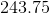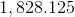The correct answer is not among the other responses.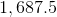Explanation:

By the Pythagorean Theorem,The similarity ratio oftoisThis can be used to find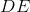: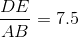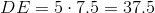The area ofis therefore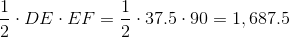### Example Question #4 : Right Triangles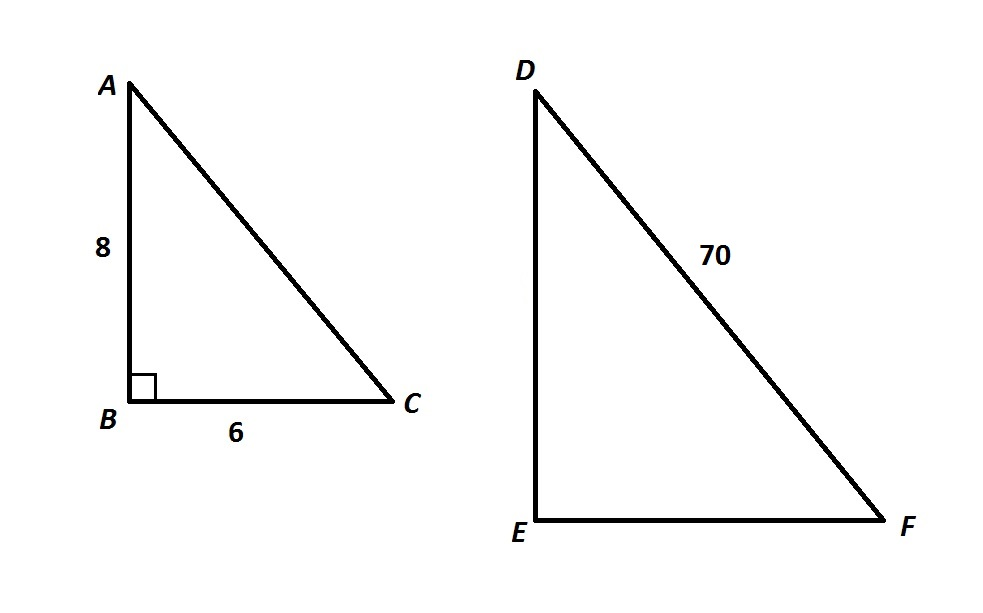Note: Figures NOT drawn to scale.

Refer to the above figure. Given that, evaluate.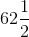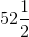Explanation:

By the Pythagorean Theorem, since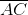is the hypotenuse of a right triangle with legs 6 and 8, its measure is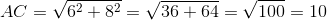.

The similarity ratio oftois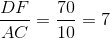.

Likewise,